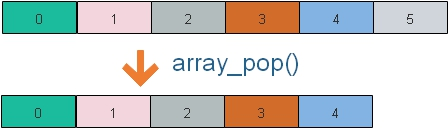# array_pop(): Taking out last element of the array

``\$output=array_pop(\$input);``Take out the last element of the array and returns the same. After applying `array_pop()` total elements of the array reduces by one. All numerical array keys remain same as last element is removed. Literal keys remain same.

Returns the shifted element ( last element ) of the array.

Here \$input is an array, \$output get the value of last element of the array.
``````\$input=array('One','Two','Three','Four');
\$output=array_pop(\$input);
echo \$output;// Output : Four
echo "<br><br>";
while (list (\$key, \$val) = each (\$input)) {
echo "\$key -> \$val <br>";
}``````
Output of above code is here( \$output gets the last element Four )
``````Four

0 -> One
1 -> Two
2 -> Three  ``````

## With Literal Keys

``````\$input=array('Fruits1' =>'Banana','Fruits2'=>'Mango','Fruits3'=>'Apple','Fruits4'=>'Grapes');
\$output=array_pop(\$input);
echo \$output;// Output : Grapes
echo "<br><br>";
while (list (\$key, \$val) = each (\$input)) {
echo "\$key -> \$val <br>";
}``````
Output is here, ( \$output gets the first element Grapes)
``````Grapes

Fruits1 -> Banana
Fruits2 -> Mango
Fruits3 -> Apple ``````

## Using end & current

By using current() we can get the element to which the present internal pointer is pointing. We can move the pointer to last element by using end.
``````\$input=array('Fruits1' =>'Banana','Fruits2'=>'Mango','Fruits3'=>'Apple','Fruits4'=>'Grapes');
end(\$input);
echo current(\$input);
echo "<br>";
\$output=array_pop(\$input);
end(\$input);
echo current(\$input); ``````
Output is here.( The last element is changed from Grapes to Apple)
``````Grapes
Apple``````

Subscribe to our YouTube Channel here

## Subscribe

* indicates required
Subscribe to plus2netplus2net.com

Post your comments , suggestion , error , requirements etc here .